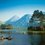# Is $\log{2} = 0$ ?

Here is a proof that $\log{2}$ = 0. This is not true but can you spot the wrong step in it.

$\displaystyle \log(1 + x) = \sum_{i = 1}^{\infty}{\dfrac{(-1)^k x^k}{k}} = x - \frac{x^2}{2} + \frac{x^3}{3} - \frac{x^4}{4} + \frac{x^5}{5} - \frac{x^6}{6} + \dots \dots \\ \text{Putting x = 1} \\ \log{2} = 1 - \frac{1}{2} + \frac{1}{3} - \frac{1}{4} + \frac{1}{5} - \frac{1}{6} + \dots \dots \\ \log{2} = 1 + \frac{1}{2} + \frac{1}{3} + \frac{1}{4} + \frac{1}{5} + \dots - 2\left(\frac{1}{2} + \frac{1}{4} + \frac{1}{6} + \frac{1}{8} + \frac{1}{10} + \dots \right) \\ \log{2} = \zeta(1) - \frac{2}{2}\left( 1 + \frac{1}{2} + \frac{1}{3} + \frac{1}{4} + \frac{1}{5} + \dots \right) \\ \log{2} = \zeta(1) - \zeta(1) \\ \log{2} = \boxed{0} \\ \textbf{Hence Proved}$Note by Rajdeep Dhingra
6 years, 3 months ago

This discussion board is a place to discuss our Daily Challenges and the math and science related to those challenges. Explanations are more than just a solution — they should explain the steps and thinking strategies that you used to obtain the solution. Comments should further the discussion of math and science.

When posting on Brilliant:

• Use the emojis to react to an explanation, whether you're congratulating a job well done , or just really confused .
• Ask specific questions about the challenge or the steps in somebody's explanation. Well-posed questions can add a lot to the discussion, but posting "I don't understand!" doesn't help anyone.
• Try to contribute something new to the discussion, whether it is an extension, generalization or other idea related to the challenge.

MarkdownAppears as
*italics* or _italics_ italics
**bold** or __bold__ bold
- bulleted- list
• bulleted
• list
1. numbered2. list
1. numbered
2. list
Note: you must add a full line of space before and after lists for them to show up correctly
paragraph 1paragraph 2

paragraph 1

paragraph 2

[example link](https://brilliant.org)example link
> This is a quote
This is a quote
    # I indented these lines
# 4 spaces, and now they show
# up as a code block.

print "hello world"
# I indented these lines
# 4 spaces, and now they show
# up as a code block.

print "hello world"
MathAppears as
Remember to wrap math in $$ ... $$ or $ ... $ to ensure proper formatting.
2 \times 3 $2 \times 3$
2^{34} $2^{34}$
a_{i-1} $a_{i-1}$
\frac{2}{3} $\frac{2}{3}$
\sqrt{2} $\sqrt{2}$
\sum_{i=1}^3 $\sum_{i=1}^3$
\sin \theta $\sin \theta$
\boxed{123} $\boxed{123}$

Sort by:

$\zeta(1) = \infty$

$\displaystyle \zeta(1) - \zeta(1) = \infty - \infty \neq 0$

- 6 years, 3 months ago

Thanks !

- 6 years, 3 months ago

Yeah, absolutely right.

- 6 years, 3 months ago

$\zeta(1)$ does not converge. So the statement $\zeta (1) - \zeta (1) =0$ is like saying $\infty - \infty = 0$ which is wrong because it is indeterminate.

- 6 years, 3 months ago

Thanks !

- 6 years, 3 months ago

Watch the limits in which the Taylor series converges. We are given that $| x | < 1$, and hence we cannot apply this to the case where $x = 1$ or $x = -1$, to find the value of $\log 2$ or $\log 0$.

Similarly, we cannot apply the Geometric Progression sum of $\frac{1}{1-x} = 1 + x + x^2 + x^3 + \ldots$ to conclude that $\frac{1}{0} = 1 + 1 + 1 + 1 + \ldots$ or that $\frac{1}{2} = 1 - 1 + 1 - 1 + \ldots$, because $x = 1, -1$ are out of the range in which the formula applies.

Staff - 6 years, 3 months ago

Thanks a Lot

- 6 years, 3 months ago

- 6 years, 3 months ago

Rajdeep did u understand all this??? It was really out of my mind!!

- 6 years, 3 months ago

I came up with this proof.

- 6 years, 3 months ago

Hey! Rajdeep are you in class 10 or 9.

- 6 years, 3 months ago

Just came in Class 9

- 6 years, 3 months ago

You are really a prodigy. How and when did you learn all this ?

- 6 years, 3 months ago

Yep! I totally agree with @Manish Dash you are a total genius. In Open proof contest can I send snapshots or a word file.

- 6 years, 3 months ago

Thanks ! You can send snapshots.

- 6 years, 3 months ago

Alternatively, this works as a proof that the harmonic series diverges.

- 6 years, 2 months ago